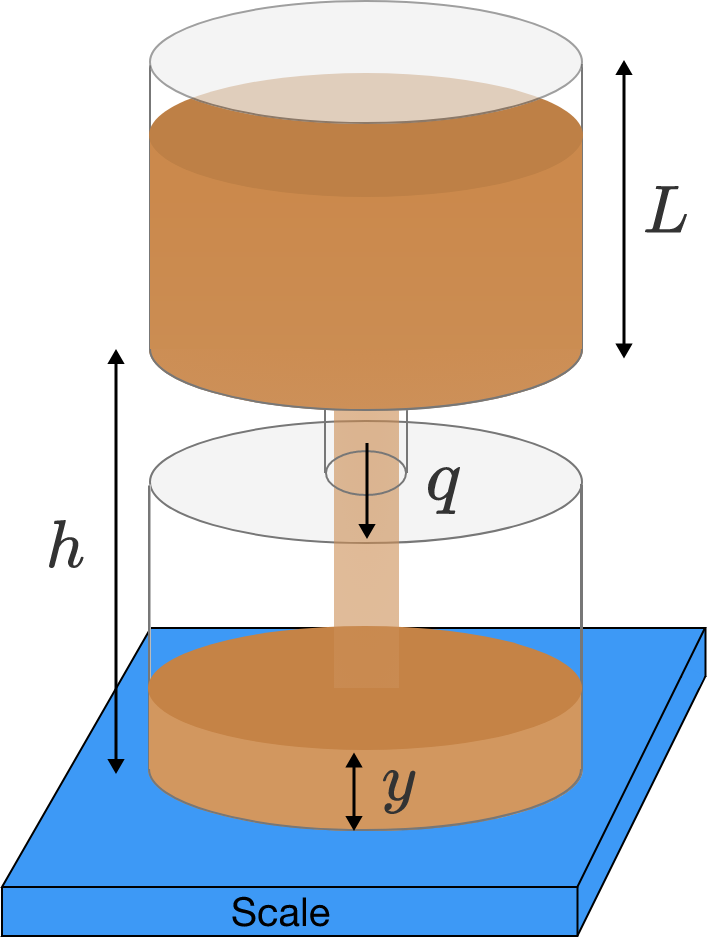# Hourglass Redux

A well-known physics problem asks whether the weight of an hourglass sitting on a scale would be the same whether all of the sand is at rest, or whether it is flowing. Usually, the answer given is yes, because of the exact balance between the "missing weight" of the sand in free fall, and the force of the sand hitting the bottom.

This well-known physics result is wrong.The hourglass above has a total mass $M$, constructed with a constant cross sectional area $A$ on the top and bottom, and a very thin section connecting them which allows the sand with density $\rho$ to flow through at a constant rate of mass flow $q$. The total amount of sand fills the bottom of the hourglass once all the sand has fallen.

What is the weight of the hourglass at steady state when the sand is flowing?

Note: You may assume that the mass of the falling sand at any given time is negligible compared to the masses at rest above and below, and that once the sand lands, it rapidly spreads out onto a flat surface.

×